## Learning Objectives

By the end of this section, you will be able to:

Before you get started, take this readiness quiz.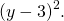In this section we will solve equations that have a variable in the radicand of a radical expression. An equation of this type is called a radical equation .

An equation in which a variable is in the radicand of a radical expression is called a radical equation .

As usual, when solving these equations, what we do to one side of an equation we must do to the other side as well. Once we isolate the radical, our strategy will be to raise both sides of the equation to the power of the index. This will eliminate the radical.

Solving radical equations containing an even index by raising both sides to the power of the index may introduce an algebraic solution that would not be a solution to the original radical equation. Again, we call this an extraneous solution as we did when we solved rational equations.

In the next example, we will see how to solve a radical equation. Our strategy is based on raising a radical with index n to the n th power. This will eliminate the radical.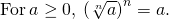• Isolate the radical on one side of the equation.
• Raise both sides of the equation to the power of the index.
• Solve the new equation.
• Check the answer in the original equation.

When we use a radical sign, it indicates the principal or positive root. If an equation has a radical with an even index equal to a negative number, that equation will have no solution.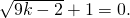Because the square root is equal to a negative number, the equation has no solution.If one side of an equation with a square root is a binomial, we use the Product of Binomial Squares Pattern when we square it.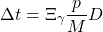Don’t forget the middle term!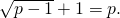When the index of the radical is 3, we cube both sides to remove the radical.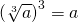Sometimes the solution of a radical equation results in two algebraic solutions, but one of them may be an extraneous solution !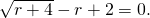When there is a coefficient in front of the radical, we must raise it to the power of the index, too.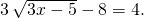If the radical equation has two radicals, we start out by isolating one of them. It often works out easiest to isolate the more complicated radical first.

In the next example, when one radical is isolated, the second radical is also isolated.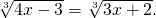Sometimes after raising both sides of an equation to a power, we still have a variable inside a radical. When that happens, we repeat Step 1 and Step 2 of our procedure. We isolate the radical and raise both sides of the equation to the power of the index again.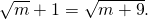We summarize the steps here. We have adjusted our previous steps to include more than one radical in the equation This procedure will now work for any radical equations.

• Isolate one of the radical terms on one side of the equation.

If yes, repeat Step 1 and Step 2 again.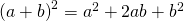As you progress through your college courses, you’ll encounter formulas that include radicals in many disciplines. We will modify our Problem Solving Strategy for Geometry Applications slightly to give us a plan for solving applications with formulas from any discipline.

• Read the problem and make sure all the words and ideas are understood. When appropriate, draw a figure and label it with the given information.
• Identify what we are looking for.
• Name what we are looking for by choosing a variable to represent it.
• Translate into an equation by writing the appropriate formula or model for the situation. Substitute in the given information.
• Solve the equation using good algebra techniques.
• Check the answer in the problem and make sure it makes sense.
• Answer the question with a complete sentence.

One application of radicals has to do with the effect of gravity on falling objects. The formula allows us to determine how long it will take a fallen object to hit the gound.

On Earth, if an object is dropped from a height of h feet, the time in seconds it will take to reach the ground is found by using the formula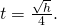It would take 2 seconds for an object dropped from a height of 64 feet to reach the ground.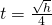Police officers investigating car accidents measure the length of the skid marks on the pavement. Then they use square roots to determine the speed , in miles per hour, a car was going before applying the brakes.

If the length of the skid marks is d feet, then the speed, s , of the car before the brakes were applied can be found by using the formula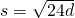Access these online resources for additional instruction and practice with solving radical equations.

• Solving an Equation Involving a Single Radical
• Solving Equations with Radicals and Rational Exponents

## Key Concepts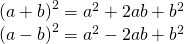## Practice Makes Perfect

In the following exercises, solve.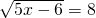no solution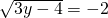In the following exercises, solve. Round approximations to one decimal place.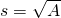## Writing Exercises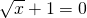ⓐ After completing the exercises, use this checklist to evaluate your mastery of the objectives of this section.ⓑ After reviewing this checklist, what will you do to become confident for all objectives?

## Radical Equation Word Problems - Examples & Practice - Expii

Radical equation word problems - examples & practice, explanations (3).

The key to solving any word problem (whether it contains a radical or not) is to translate the problem from words into math .

That's the biggest step in word problems. Once you've translated the information into numbers, you solve the equation the same way as always.

Let's look at an example to see how this approach works when radicals are involved.

Hiroki is calculating the speed of a tsunami. The formula is speed=√9.8d where d is ocean depth in meters. In this case, the ocean was 1500 meters deep. How fast was the wave (in m/s2)?

So, in this problem (as with many problems involving radicals), the formula to use has already been given to us. We are interested in the speed of the wave, and we've been given the depth (d). So, let's start by plugging that value in : speed=√9.8(1500) Let's solve this problem.

How fast was the wave (m/s2)?

## Related Lessons

Example walk through: word problem involving radicals.

The key to word problems is in the translation. Let's walk through the problem below and solve it.

Lori recently purchased a square painting with an area of 780 cm2. How much wood does Lori need to make the frame? Don't worry about the width and height of the wood, just find the length.

First, find out the essential information in the problem.

A square painting has area 780cm2, what is its side length?

What is the relationship between side length and area of a square? Area=side length×side length

Denote side length as x for simplicity, plug in the numbers we have: 780cm2=x×x Solve for x: 780=x2x=√78x≈27.93

Lastly, note that the question is asking how much wood Lori needs to build the frame. A square has 4 sides so we have to multiply 27.93cm by 4.

In total, Lori needs at least 27.93cm×4=111.72cm of wood.

Let's look at another problem!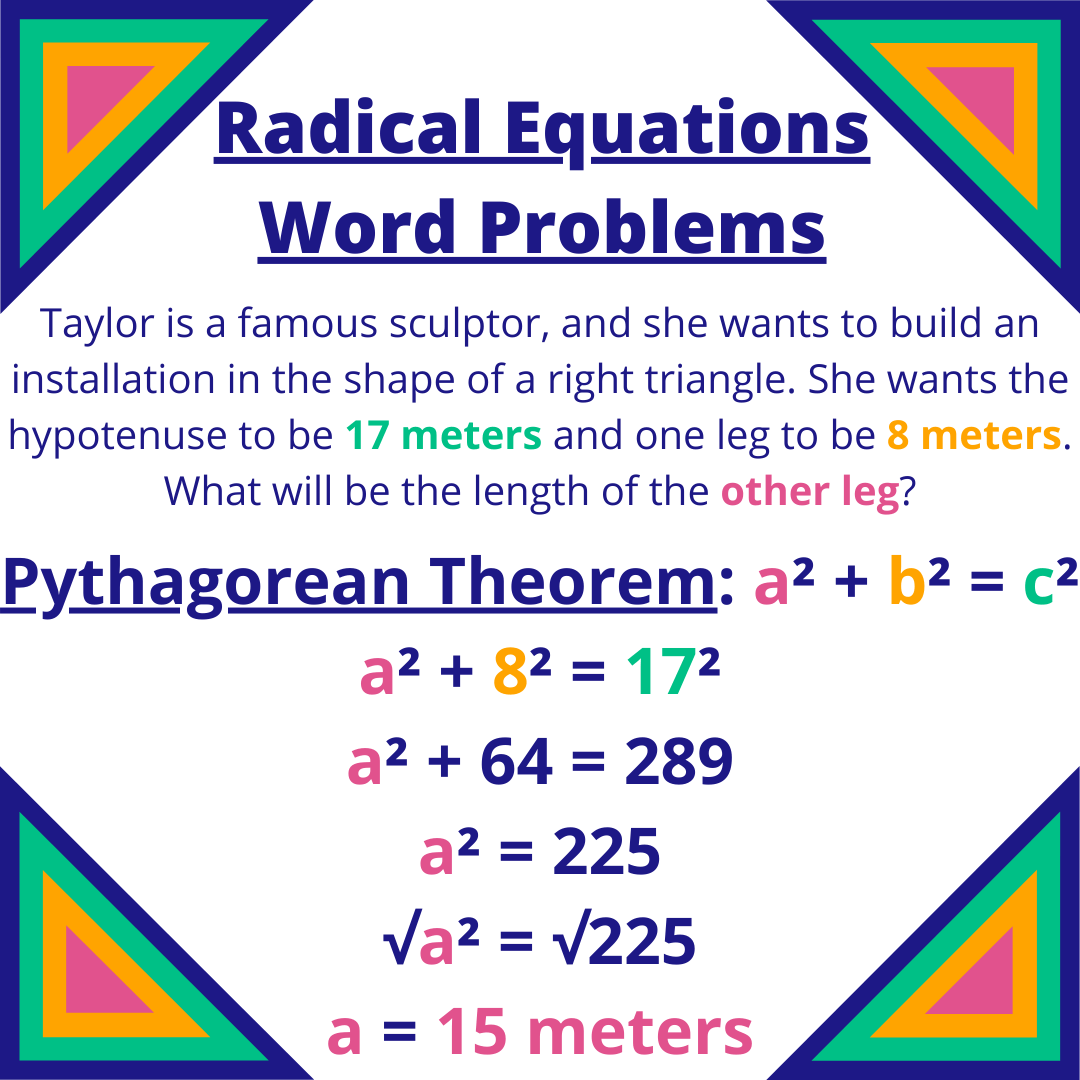Image source: By Caroline Kulczycky## (Video) Problem Solving with Radical Expressions

by Deanna Green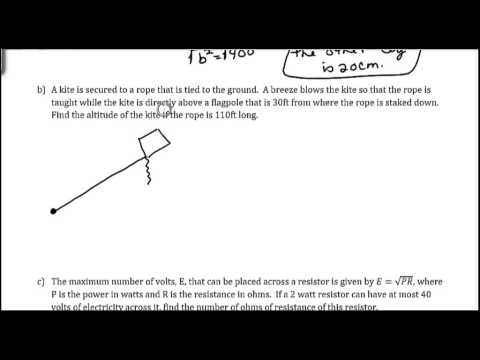This video by Deanna Green works through word problems with radicals.

These problems usually focus around the Pythagorean Theorem . A helpful trick always is to draw a simple picture of what's going on. Then fill in lengths or angles as the problem states.

Let's work through one of the examples she looks at.

A kite is secured to a rope that is tied to the ground. A breeze blows the kite so that the rope is taught while the kite is directly above a flagpole that is 30 ft from where the rope is staked down. Find the altitude of the kite if the rope is 110 ft long.

The first step is to draw a diagram.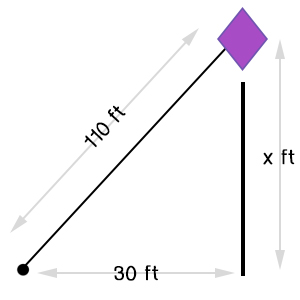Image source: by Alex Federspiel

The Pythagorean Theorem states:

The length of the hypotenuse (diagonal line) is equal to the square root of the square of the sides added.

As an equation:

where c is the hypotenuse and a and b are the other sides.

Start by plugging in all the information we know.

110=√302+x2

You cannot split up radicals with a sum underneath into two separate radicals. So, to solve this, we want to take the square of both sides.

(110)2=(√302+x2)212100=302+x212100−302=x212100−900=x211200=x2√11200=√x2105.83≈x

You may need to review the lesson on getting rid of the x2 in with this lesson .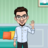This will provide examples and solution on how to solve problems involving radical expressions.

## Recommended## More Related Content

What's hot.## What's hot ( 20 )## Similar to Solving Problems Involving Radicals## Similar to Solving Problems Involving Radicals ( 20 )## Recently uploaded ( 20 )• 1. MATHEMATICS 9 Quarter 2: Module 9 Week 9 PROBLEMS INVOLVING RADICALS
• 2. Problems Involving Radicals Some of the important applications of radicals are problems involving the Pythagorean Theorem and the distance formula. Radicals are also often used in science.
• 3. Ancient Greek mathematicians studied a number of properties of right triangles. One result states that in a right triangle, the sum of the squares of the legs is equal to the square of the hypotenuse. This property is called the Pythagorean Theorem, in honor of Pythagoras (circa 590 B.C.). In symbols, the theorem says that (hypotenuse)2 = (leg)2 + (leg)2. From this equation, the hypotenuse c is equal to 𝒉𝒚𝒑𝒐𝒕𝒆𝒏𝒖𝒔𝒆 = (𝒍𝒆𝒈)𝟐+(𝒍𝒆𝒈)𝟐 leg leg hypotenuse
• 4. STEPS IN SOLVING WORD PROBLEMS 1. Read and analyze the problem carefully. 2. Illustrate the problem. 3. Develop the plan to solve it. 4. Solve the problem 5. Looking back.
• 5. Examples and Solutions
• 6. Example 1: Find the length of the hypotenuse of a right triangle whose legs are 5 cm and 12 cm. Solutions: The hypotenuse of a right triangle is the side opposite the right angle. the other sides are the legs. Let c = hypotenuse 𝑐 = (5) 2+ 12 2 𝑐 = 25 + 144 𝑐 = 169 𝒄 = 𝟏𝟑 5 cm 12 cm c Therefore, the length of the hypotenuse of the triangle is 13 cm.
• 7. Example 2: A small plane and a helicopter left an airport at the same time. After several minutes, the plane is 7 miles due north of the airport while the helicopter is 5 miles due east of the airport. How far apart are they at that time? Solutions: Let x = distance between the plane and helicopter 𝑥 = (7) 2+ 5 2 𝑥 = 49 + 25 𝑥 = 74 𝒙 ≈ 𝟖. 𝟔 7 mi 5 mi x Plane (North) Helicopter (East) Airport Therefore, the distance between the plane and helicopter that time is approximately 8.6 miles.
• 8. Example 3: Find the diagonal of a square whose area is 40 square meters. Solutions: The area of a square with side s is s2. Moreover, the diagonal d forms a right triangle with two sides each of whose length is s. 𝑑 = 𝑠 2 + 𝑠2 𝑑 = 2𝑠2 𝑑 = 2 40 𝑑 = 80 𝒙 = 4 𝟓 d s s Therefore, the length of the diagonal of the square is 4 𝟓 m.
• 9. Example 4: Find the area of a triangle with sides 8 cm, 9 cm, and 11 cm, respectively. Solutions: The area of a triangle can be found using the formula 𝑨 = 𝒔(𝒔 − 𝒂)(𝒔 − 𝒃)(𝒔 − 𝒄) where A is the area of a triangle, a, b, and c are the lengths of the sides, and 𝒔 = 𝟏 𝟐 𝒂 + 𝒃 + 𝒄 . Since, a = 8, b = 9, and c = 11 𝑠 = 1 2 (8 + 9 + 11) 𝑠 = 1 2 28 𝒔 = 𝟏𝟒 Heron’s Formula 𝑨 = 𝒔(𝒔 − 𝒂)(𝒔 − 𝒃)(𝒔 − 𝒄)
• 10. Example 4: Find the area of a triangle with sides 8 cm, 9 cm, and 11 cm, respectively. Solutions: And the area is, 𝐴 = 𝑠(𝑠 − 𝑎)(𝑠 − 𝑏)(𝑠 − 𝑐) 𝐴 = 14(14 − 8)(14 − 9)(14 − 11) 𝐴 = 14(6)(5)(3) 𝐴 = 1260 𝐴 = (36)(35) 𝑨 = 𝟔 𝟑𝟓 Therefore, the area of the triangle is 𝟔 𝟑𝟓.
• 11. Example 5: Galileo Galilei performed many experiments on freely falling bodies at the Leaning Tower of Pisa. His experiments led to the formulation of relation between the velocity of a freely falling body and the distance travelled by the body. This relationship is expressed by the formula 𝒗 = 𝟐𝒈𝒉 where v is the velocity of the object in ft/s, h is the distance and g is the acceleration due to the gravity whose approximate value on Earth is 32 ft/s2. Find the velocity of a stone after it has fallen 15 ft.
• 12. Continuation: Example 5: Find the velocity of a stone after it has fallen 15 ft. Solutions: The distance (h) is 15 ft and the acceleration due to gravity is (g) is 32 ft/s2, hence, the velocity (v) is: 𝑣 = 2𝑔ℎ 𝑣 = 2 32 ൗ 𝑓𝑡 𝑠2 (15𝑓𝑡) 𝑣 = 960 ൗ 𝑓𝑡2 𝑠2 ≈ 𝟑𝟎. 𝟗𝟖 Τ 𝒇𝒕 𝒔 Therefore, the velocity of the stone is approximately 𝟑𝟎. 𝟗𝟖 Τ 𝒇𝒕 𝒔.
• 13. Example 6: The square root of the sum of a number and 5 is 3. Find the number. Solutions: Let x be the number. Checking: x = 4 Equation: 𝑥 + 5 = 3 𝑥 + 5 = 3 Solve for x. 4 + 5 = 3 𝑥 + 5 = 3 9 = 3 𝑥 + 5 2 = 3 2 3 = 3 TRUE 𝑥 + 5 = 9 𝑥 = 9 − 5 𝒙 = 𝟒 Therefore, the number is 4.
• 14. Example 7: The square root of the difference between a number and 5, plus the square root of the number is equal to 5. Find the number. Solutions: Let x be the number. Checking: x = 9 Equation: 𝑥 − 5 + 𝑥 = 5 𝑥 − 5 + 𝑥 = 5 Solve for x. 9 − 5 + 9 = 5 𝑥 − 5 = 5 − 𝑥 4 + 3 = 5 𝑥 − 5 2 = 5 − 𝑥 2 2+3 = 5 𝑥 − 5 = 25 − 10 𝑥 + 𝑥 5 = 5 TRUE 10 𝑥 = 30 𝑥 = 3 𝒙 = 𝟗 Therefore, the number is 9.• My Preferences
• Study Guides
• Linear Equations
• Quiz: Linear Equations
• Quiz: Formulas
• Absolute Value Equations
• Quiz: Absolute Value Equations
• Linear Inequalities
• Quiz: Linear Inequalities
• Compound Inequalities
• Quiz: Compound Inequalities
• Absolute Value Inequalities
• Quiz: Absolute Value Inequalities
• Rectangular Coordinate System
• Quiz: Rectangular Coordinate System
• Distance Formula
• Quiz: Distance Formula
• Midpoint Formula
• Quiz: Midpoint Formula
• Slope of a Line
• Quiz: Slope of a Line
• Slopes of Parallel and Perpendicular Lines
• Quiz: Slopes of Parallel and Perpendicular Lines
• Equations of Lines
• Quiz: Equations of Lines
• Graphs of Linear Inequalities
• Quiz: Graphs of Linear Inequalities
• Linear Equations: Solutions Using Graphing with Two Variables
• Quiz: Linear Equations: Solutions Using Graphing with Two Variables
• Linear Equations: Solutions Using Substitution with Two Variables
• Quiz: Linear Equations: Solutions Using Substitution with Two Variables
• Linear Equations: Solutions Using Elimination with Two Variables
• Quiz: Linear Equations: Solutions Using Elimination with Two Variables
• Linear Equations: Solutions Using Matrices with Two Variables
• Quiz: Linear Equations: Solutions Using Matrices with Two Variables
• Linear Equations: Solutions Using Determinants with Two Variables
• Quiz: Linear Equations: Solutions Using Determinants with Two Variables
• Linear Inequalities: Solutions Using Graphing with Two Variables
• Quiz: Linear Inequalities: Solutions Using Graphing with Two Variables
• Quiz: Linear Equations: Solutions Using Elimination with Three Variables
• Linear Equations: Solutions Using Elimination with Three Variables
• Linear Equations: Solutions Using Matrices with Three Variables
• Quiz: Linear Equations: Solutions Using Matrices with Three Variables
• Linear Equations: Solutions Using Determinants with Three Variables
• Quiz: Linear Equations: Solutions Using Determinants with Three Variables
• Quiz: Adding and Subtracting Polynomials
• Multiplying Polynomials
• Quiz: Multiplying Polynomials
• Special Products of Binomials
• Quiz: Special Products of Binomials
• Dividing Polynomials
• Quiz: Dividing Polynomials
• Synthetic Division
• Quiz: Synthetic Division
• Greatest Common Factor
• Quiz: Greatest Common Factor
• Difference of Squares
• Quiz: Difference of Squares
• Sum or Difference of Cubes
• Quiz: Sum or Difference of Cubes
• Trinomials of the Form x^2 + bx + c
• Quiz: Trinomials of the Form x^2 + bx + c
• Trinomials of the Form ax^2 + bx + c
• Quiz: Trinomials of the Form ax^2 + bx + c
• Square Trinomials
• Quiz: Square Trinomials
• Factoring by Regrouping
• Quiz: Factoring by Regrouping
• Summary of Factoring Techniques
• Solving Equations by Factoring
• Quiz: Solving Equations by Factoring
• Examples of Rational Expressions
• Quiz: Examples of Rational Expressions
• Simplifying Rational Expressions
• Quiz: Simplifying Rational Expressions
• Multiplying Rational Expressions
• Quiz: Multiplying Rational Expressions
• Dividing Rational Expressions
• Quiz: Dividing Rational Expressions
• Adding and Subtracting Rational Expressions
• Quiz: Adding and Subtracting Rational Expressions
• Complex Fractions
• Quiz: Complex Fractions
• Solving Rational Equations
• Quiz: Solving Rational Equations
• Proportion, Direct Variation, Inverse Variation, Joint Variation
• Quiz: Proportion, Direct Variation, Inverse Variation, Joint Variation
• Graphing Rational Functions
• Quiz: Graphing Rational Functions
• Basic Definitions
• Quiz: Basic Definitions
• Function Notation
• Quiz: Function Notation
• Compositions of Functions
• Quiz: Compositions of Functions
• Algebra of Functions
• Quiz: Algebra of Functions
• Inverse Functions
• Quiz: Inverse Functions
• Polynomial Function
• Quiz: Polynomial Function
• Remainder Theorem
• Quiz: Remainder Theorem
• Factor Theorem
• Quiz: Factor Theorem
• Zeros of a Function
• Quiz: Zeros of a Function
• Rational Zero Theorem
• Quiz: Rational Zero Theorem
• Graphing Polynomial Functions
• Quiz: Graphing Polynomial Functions
• Rational Exponents
• Quiz: Rational Exponents
• Complex Numbers
• Quiz: Complex Numbers
• Quiz: Solving Quadratics by Factoring
• Solving Quadratics by the Square Root Property
• Quiz: Solving Quadratics by the Square Root Property
• Solving Quadratics by Completing the Square
• Quiz: Solving Quadratics by Completing the Square
• Solving Equations in Quadratic Form
• Quiz: Solving Equations in Quadratic Form
• The Four Conic Sections
• Quiz: The Four Conic Sections
• Quiz: Circle
• Quiz: Parabola
• Quiz: Ellipse
• Quiz: Hyperbola
• Systems of Equations Solved Algebraically
• Quiz: Systems of Equations Solved Algebraically
• Systems of Equations Solved Graphically
• Quiz: Systems of Equations Solved Graphically
• Systems of Inequalities Solved Graphically
• Exponential Functions
• Quiz: Exponential Functions
• Logarithmic Functions
• Quiz: Logarithmic Functions
• Properties of Logarithms
• Quiz: Properties of Logarithms
• Exponential and Logarithmic Equations
• Quiz: Exponential and Logarithmic Equations
• Definition and Examples of Sequences
• Quiz: Definition and Examples of Sequences
• Arithmetic Sequence
• Quiz: Arithmetic Sequence
• Arithmetic Series
• Quiz: Arithmetic Series
• Geometric Sequence
• Quiz: Geometric Sequence
• Geometric Series
• Quiz: Geometric Series
• Summation Notation
• Quiz: Summation Notation
• Quiz: Factorials
• Binomial Coefficients and the Binomial Theorem
• Quiz: Binomial Coefficients and the Binomial Theorem
• Permutations
• Quiz: Permutations
• Combinations
• Quiz: Combinations
• General Strategy
• Simple Interest
• Quiz: Simple Interest
• Compound Interest
• Quiz: Compound Interest
• Quiz: Mixture
• Quiz: Motion
• Arithmetic/Geometric Series
• Quiz: Arithmetic/Geometric Series
• Algebra II Quiz

A radical equation is an equation in which a variable is under a radical. To solve a radical equation:

Isolate the radical expression involving the variable. If more than one radical expression involves the variable, then isolate one of them.

Raise both sides of the equation to the index of the radical.

If there is still a radical equation, repeat steps 1 and 2; otherwise, solve the resulting equation and check the answer in the original equation.

By raising both sides of an equation to a power, some solutions may have been introduced that do not make the original equation true. These solutions are called extraneous solutions.Raise both sides to the index of the radical; in this case, square both sides.This quadratic equation now can be solved either by factoring or by applying the quadratic formula.Now, check the results.Isolate one of the radical expressions.This can be solved either by factoring or by applying the quadratic formula.Check the solutions.So x = 10 is not a solution.The only solution is x = 2.Isolate the radical involving the variable.Since radicals with odd indexes can have negative answers, this problem does have solutions. Raise both sides of the equation to the index of the radical; in this case, cube both sides.The check of the solution x = –15 is left to you.

Previous Quiz: Solving Equations in Quadratic Form

• Online Quizzes for CliffsNotes Algebra II Quick Review, 2nd Edition

Removing #book# from your Reading List will also remove any bookmarked pages associated with this title.

Are you sure you want to remove #bookConfirmation# and any corresponding bookmarks?

## Module 4: Equations and Inequalities

Equations with radicals and rational exponents, learning outcomes.

• Solve a radical equation, identify extraneous solution.
• Solve an equation with rational exponents.

Radical equations are equations that contain variables in the radicand (the expression under a radical symbol), such as

Radical equations may have one or more radical terms and are solved by eliminating each radical, one at a time. We have to be careful when solving radical equations as it is not unusual to find extraneous solutions , roots that are not, in fact, solutions to the equation. These solutions are not due to a mistake in the solving method, but result from the process of raising both sides of an equation to a power. Checking each answer in the original equation will confirm the true solutions.

## A General Note: Radical Equations

An equation containing terms with a variable in the radicand is called a radical equation .

## How To: Given a radical equation, solve it

• Isolate the radical expression on one side of the equal sign. Put all remaining terms on the other side.
• If the radical is a square root, then square both sides of the equation. If it is a cube root, then raise both sides of the equation to the third power. In other words, for an n th root radical, raise both sides to the n th power. Doing so eliminates the radical symbol.
• Solve the resulting equation.
• If a radical term still remains, repeat steps 1–2.
• Check solutions by substituting them into the original equation.

## Example: Solving an Equation with One Radical

Solve $\sqrt{15 - 2x}=x$.

The radical is already isolated on the left side of the equal sign, so proceed to square both sides.

We see that the remaining equation is a quadratic. Set it equal to zero and solve.

The proposed solutions are $x=-5$ and $x=3$. Let us check each solution back in the original equation. First, check $x=-5$.

This is an extraneous solution. While no mistake was made solving the equation, we found a solution that does not satisfy the original equation.

Check $x=3$.

The solution is $x=3$.

Solve the radical equation: $\sqrt{x+3}=3x - 1$

$x=1$; extraneous solution $x=-\frac{2}{9}$

Solve $\sqrt{2x+3}+\sqrt{x - 2}=4$.

As this equation contains two radicals, we isolate one radical, eliminate it, and then isolate the second radical.

Use the perfect square formula to expand the right side: ${\left(a-b\right)}^{2}={a}^{2}-2ab+{b}^{2}$.

Now that both radicals have been eliminated, set the quadratic equal to zero and solve.

The proposed solutions are $x=3$ and $x=83$. Check each solution in the original equation.

One solution is $x=3$.

Check $x=83$.

The only solution is $x=3$. We see that $x=83$ is an extraneous solution.

Solve the equation with two radicals: $\sqrt{3x+7}+\sqrt{x+2}=1$.

$x=-2$; extraneous solution $x=-1$

## Solve Equations With Rational Exponents

Rational exponents are exponents that are fractions, where the numerator is a power and the denominator is a root. For example, ${16}^{\frac{1}{2}}$ is another way of writing $\sqrt{16}$; ${8}^{\frac{1}{3}}$ is another way of writing $\text{ }\sqrt{8}$. The ability to work with rational exponents is a useful skill as it is highly applicable in calculus.

We can solve equations in which a variable is raised to a rational exponent by raising both sides of the equation to the reciprocal of the exponent. The reason we raise the equation to the reciprocal of the exponent is because we want to eliminate the exponent on the variable term, and a number multiplied by its reciprocal equals 1. For example, $\frac{2}{3}\left(\frac{3}{2}\right)=1$, $3\left(\frac{1}{3}\right)=1$, and so on.

## A General Note: Rational Exponents

A rational exponent indicates a power in the numerator and a root in the denominator. There are multiple ways of writing an expression, a variable, or a number with a rational exponent:

## Example: Evaluating a Number Raised to a Rational Exponent

Evaluate ${8}^{\frac{2}{3}}$.

Whether we take the root first or the power first depends on the number. It is easy to find the cube root of 8, so rewrite ${8}^{\frac{2}{3}}$ as ${\left({8}^{\frac{1}{3}}\right)}^{2}$.

Evaluate ${64}^{-\frac{1}{3}}$.

$\frac{1}{4}$

## Example: Solve the Equation Including a Variable Raised to a Rational Exponent

Solve the equation in which a variable is raised to a rational exponent: ${x}^{\frac{5}{4}}=32$.

The way to remove the exponent on x is by raising both sides of the equation to a power that is the reciprocal of $\frac{5}{4}$, which is $\frac{4}{5}$.

Solve the equation ${x}^{\frac{3}{2}}=125$.

$25$

## Example: Solving an Equation Involving Rational Exponents and Factoring

Solve $3{x}^{\frac{3}{4}}={x}^{\frac{1}{2}}$.

This equation involves rational exponents as well as factoring rational exponents. Let us take this one step at a time. First, put the variable terms on one side of the equal sign and set the equation equal to zero.

Now, it looks like we should factor the left side, but what do we factor out? We can always factor the term with the lowest exponent. Rewrite ${x}^{\frac{1}{2}}$ as ${x}^{\frac{2}{4}}$. Then, factor out ${x}^{\frac{2}{4}}$ from both terms on the left.

Where did ${x}^{\frac{1}{4}}$ come from? Remember, when we multiply two numbers with the same base, we add the exponents. Therefore, if we multiply ${x}^{\frac{2}{4}}$ back in using the distributive property, we get the expression we had before the factoring, which is what should happen. We need an exponent such that when added to $\frac{2}{4}$ equals $\frac{3}{4}$. Thus, the exponent on x in the parentheses is $\frac{1}{4}$.

Let us continue. Now we have two factors and can use the zero factor theorem.

The two solutions are $x=0$, $x=\frac{1}{81}$.

Solve: ${\left(x+5\right)}^{\frac{3}{2}}=8$.

$-1$

## Contribute!

• Question ID 2118. Authored by : Lawrence Morales. License : CC BY: Attribution . License Terms : IMathAS Community License CC- BY + GPL
• Question ID 2608, 2552. Authored by : Greg Langkamp. License : CC BY: Attribution . License Terms : IMathAS Community License CC- BY + GPL
• Question ID 38391, 38406. Authored by : Tyler Wallace. License : CC BY: Attribution . License Terms : IMathAS Community License CC- BY + GPL
• College Algebra. Authored by : OpenStax College Algebra. Provided by : OpenStax. Located at : http://cnx.org/contents/9b08c294-057f-4201-[email protected]:1/Preface . License : CC BY: Attribution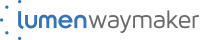#### IMAGES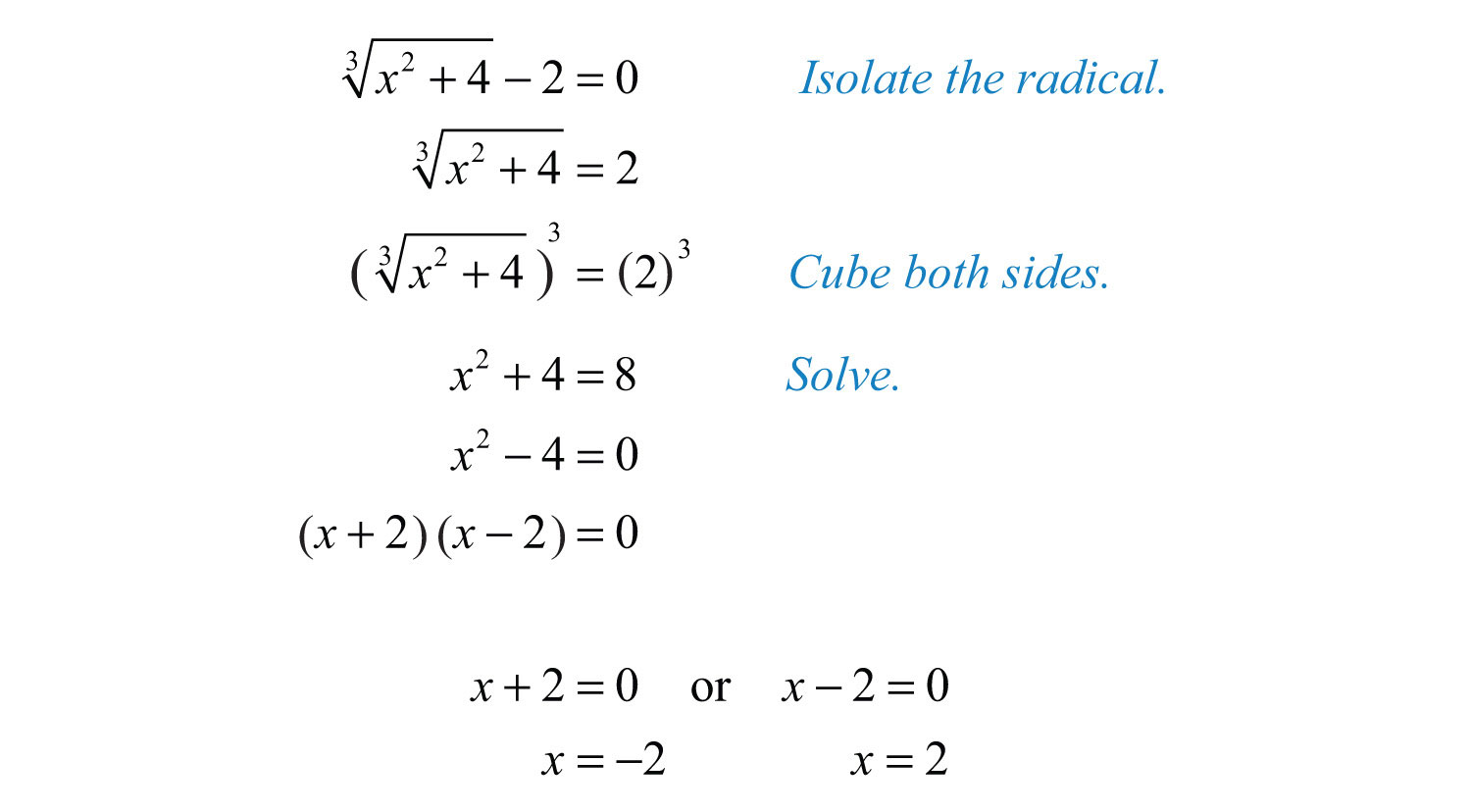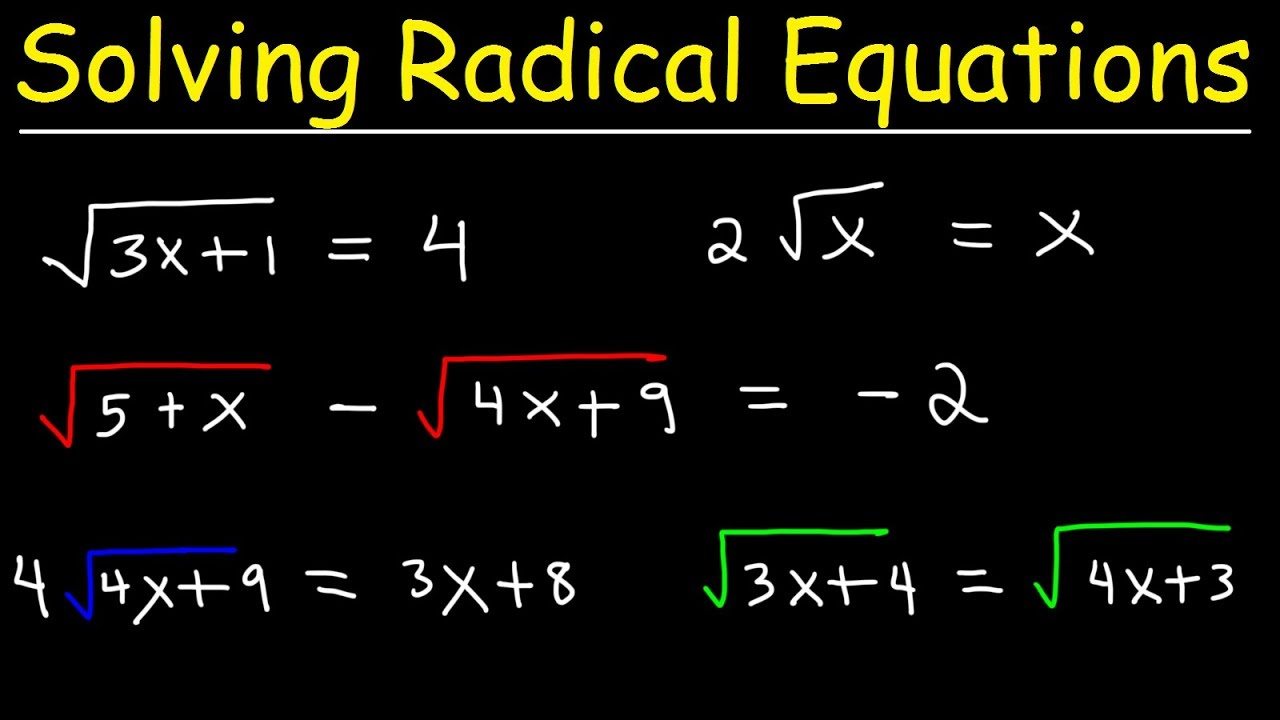4. Radical Equations Word Problems Examples5. 50 Solve Radical Equations Worksheet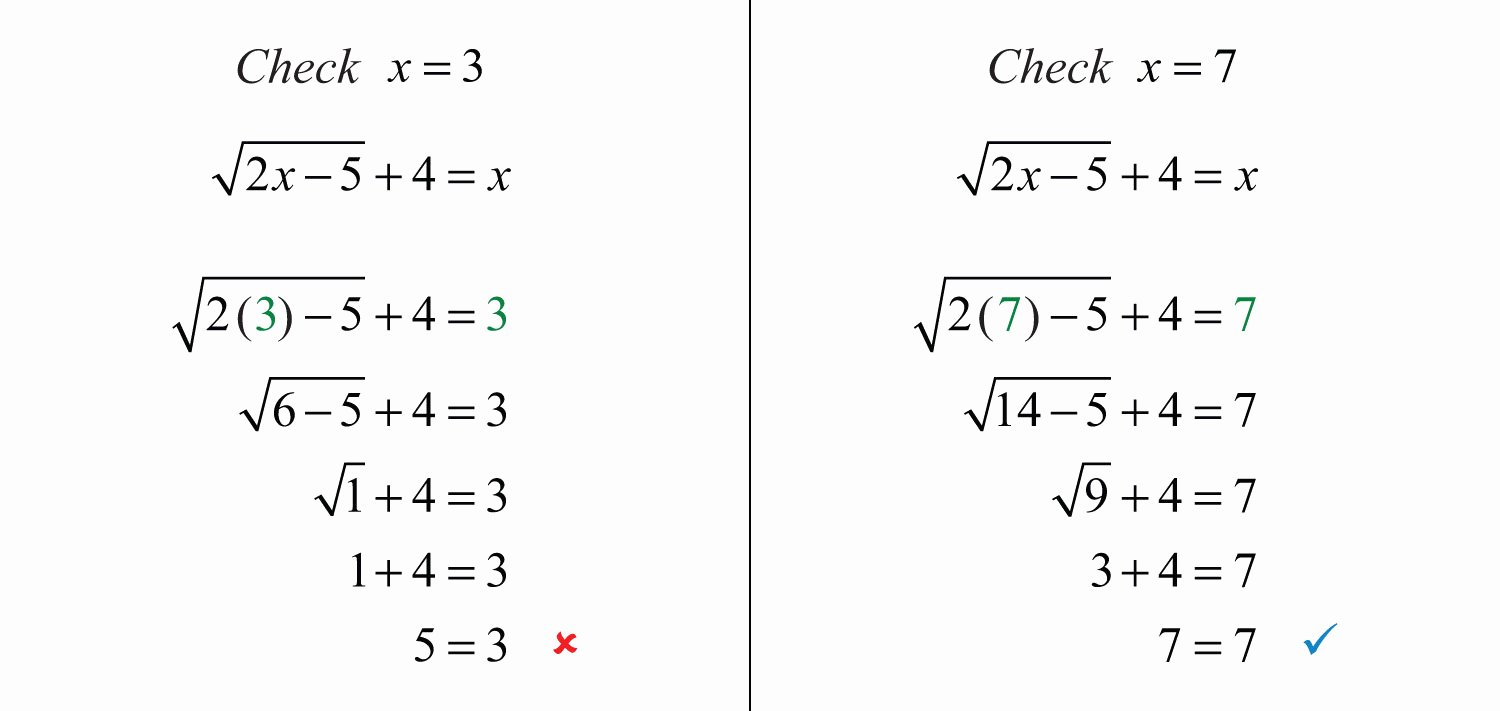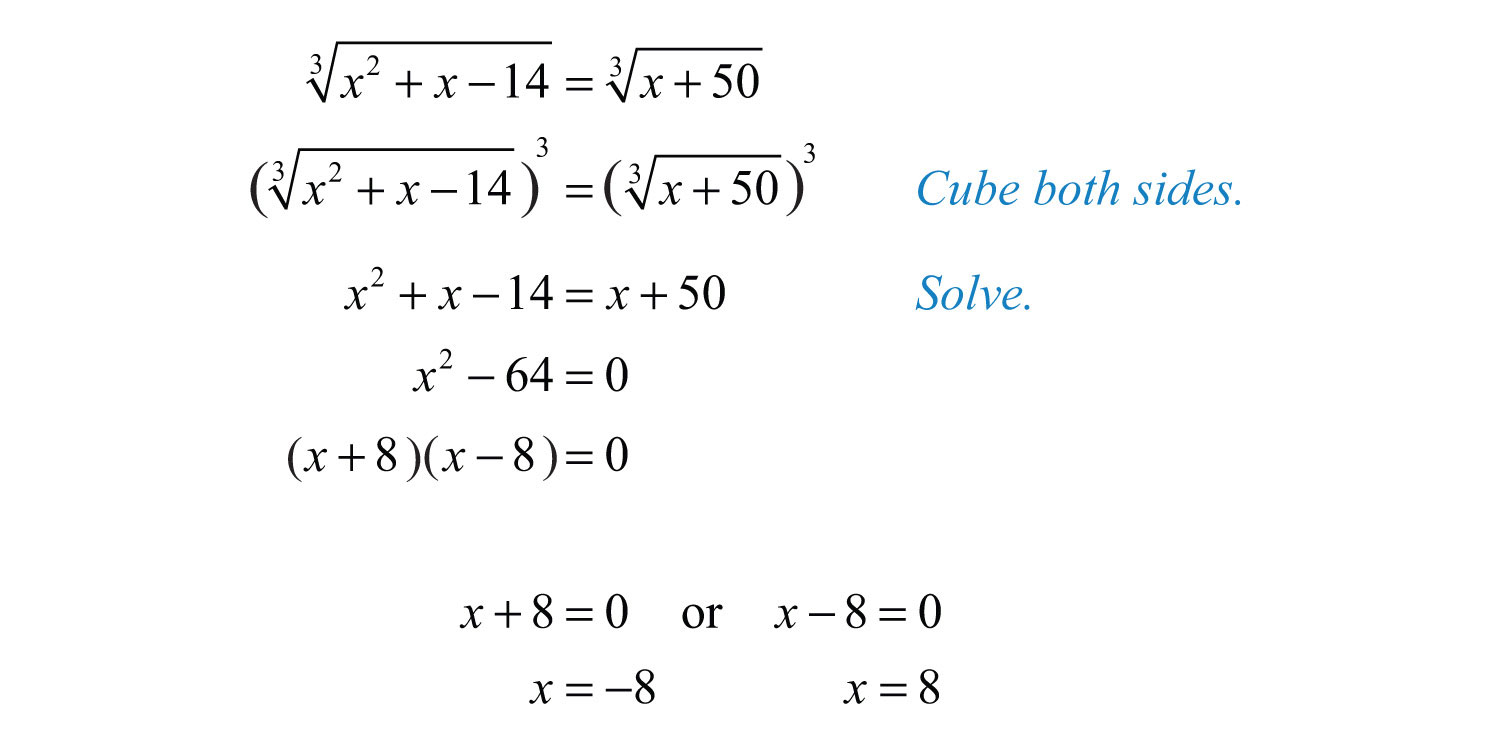#### VIDEO

3. Solving Radical Equations With Square Roots, Cube Roots, Two Radicals, Fractions, Rational Exponents

6. Solving Radical Equations ( Easy, Average & Difficult Examples )

1. Solve for Y in Terms of X?

According to the University of Regina, another way to express solving for y in terms of x is solving an equation for y. The solution is not a numerical value; instead, it is an expression equal to y involving the variable x. An example prob...

Whether you love math or suffer through every single problem, there are plenty of resources to help you solve math equations. Skip the tutor and log on to load these awesome websites for a fantastic free equation solver or simply to find an...

3. What Are the Six Steps of Problem Solving?

The six steps of problem solving involve problem definition, problem analysis, developing possible solutions, selecting a solution, implementing the solution and evaluating the outcome. Problem solving models are used to address issues that...

4. How to Solve Basic Word Problems Involving Radical Equations

Solving a Basic Word Problem Involving Radical Equations: Unknown Inside the Radical Example ... Find the length, L, of the pendulum if 2 π is the

Solve Radical Equations · Isolate the radical on one side of the equation. · Raise both sides of the equation to the power of the index. · Solve the new equation.

An equation in which a variable is in the radicand of a radical expression is called a radical equation. As usual, when solving these equations

7. Solving Word Problems Involving Radicals

To solve a radical equation: 1. Isolate the radical expression involving the variable. ... 2. Raise both sides of the equation to the index

Radical Equations Word Problems. Taylor is a famous sculptor, and she wants to build. Image source: By Caroline Kulczycky. Report. Share. 2. Like.

Identify extraneous solutions. Solve real-life problems involving radical equations. Solving Radical Equations. A radical equation is an equation that contains

STEPS IN SOLVING WORD PROBLEMS 1. Read and analyze the problem carefully. 2. Examples and Solutions. Example 1: Find the

Solving Radical Equations · 1) Isolate the radical symbol on one side of the equation · 2) Square both sides of the equation to eliminate the radical symbol · 3)